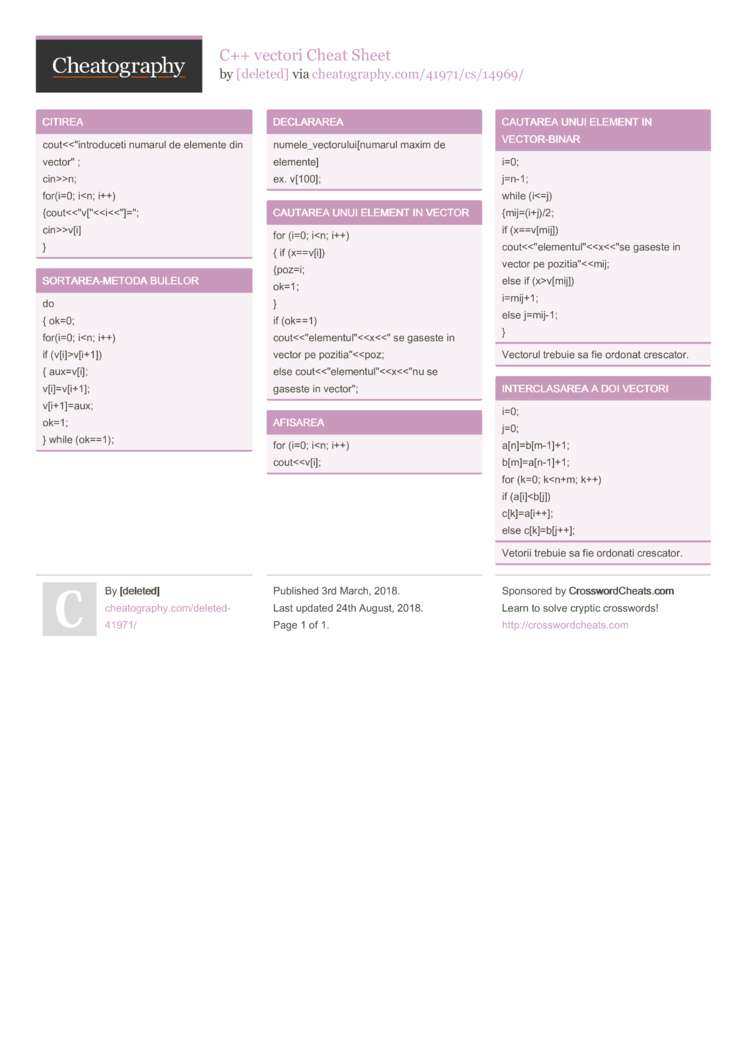# C++ vectori Cheat Sheet by [deleted]

### CITIREA

 cout<<­"­int­rod­uceti numarul de elemente din vector­" ; cin>>n; for(i=0; i

### SORTAR­EA-­METODA BULELOR

 do { ok=0; for(i=0; i­v[i+1]) { aux=v[i]; v[i]=v­[i+1]; v[i+1]­=aux; ok=1; } while (ok==1);

### DECLARAREA

 numele­_ve­cto­rul­ui[­numarul maxim de elemente] ex. v;

### CAUTAREA UNUI ELEMENT IN VECTOR

 for (i=0; i

### AFISAREA

 for (i=0; i

### CAUTAREA UNUI ELEMENT IN VECTOR­-BINAR

 i=0; j=n-1; while (i<=j) {mij=(­i+j)/2; if (x==v[­mij]) cout<<­"­ele­men­tul­"­<­v[mij]) i=mij+1; else j=mij-1; }
Vectorul trebuie sa fie ordonat crescator.

### INTERC­LASAREA A DOI VECTORI

 i=0; j=0; a[n]=b­[m-­1]+1; b[m]=a­[n-­1]+1; for (k=0; k
Vetorii trebuie sa fie ordonati crescator.1 Page
//media.cheatography.com/storage/thumb/deleted-41971_c-vectori.750.jpg

PDF (recommended)